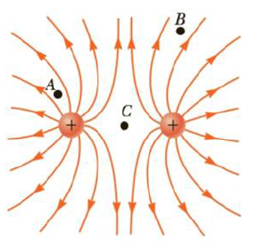Chapter 15.4, Problem 15.6QQ

Chapter
Section
Textbook Problem

Rank the magnitudes of the electric field at points A, B, and C in Figure 15.15, with the largest magnitude first.(a) A, B, C (b) A, C, B (c) C, A, B (d) The answer can’t be determined by visual inspection.Figure 15.15 The electric field lines for two positive point charges. The points A, B, and C are discussed in Quick Quiz 15.6.

To determine
The electric fields at points A, B and C.

Explanation

The strength of the electric field at the points can be identified by noting the closeness of the field lines due to charges.

Conclusion:

At point A, the field lines are the closest. At point B, the field lines are closer. At point C, the field lines are negligible. Thus, option (a) is correct.

The strength of electric field at point B is greater than that at point C because the more field lines are present at point B...

Still sussing out bartleby?

Check out a sample textbook solution.

See a sample solution

The Solution to Your Study Problems

Bartleby provides explanations to thousands of textbook problems written by our experts, many with advanced degrees!

Get Started